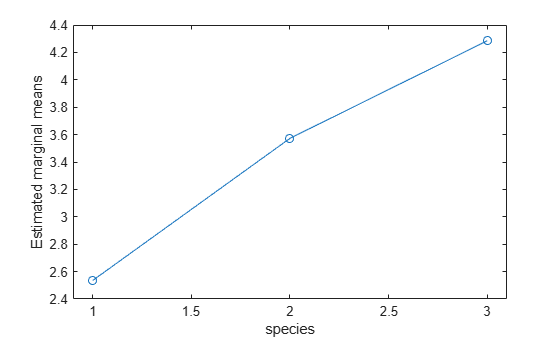# plotprofile

Class: RepeatedMeasuresModel

Plot expected marginal means with optional grouping

## Syntax

``plotprofile(rm,X)``
``plotprofile(rm,Name,Value)``
``H = plotprofile(___)``

## Description

example

````plotprofile(rm,X)` plots the expected marginal means computed from the repeated measures model `rm` as a function of the variable `X`.```

example

````plotprofile(rm,Name,Value)` plots the expected marginal means computed from the repeated measures model `rm` with additional options specified by one or more `Name,Value` pair arguments.For example, you can specify the factors to group by or change the line colors.```
````H = plotprofile(___)` returns handles, `H`, to the plotted lines.```

## Input Arguments

expand all

Repeated measures model, returned as a `RepeatedMeasuresModel` object.

For properties and methods of this object, see `RepeatedMeasuresModel`.

Name of a between-subjects or within-subjects factor, specified as a character vector or string scalar.

For example, if you want to plot the marginal means as a function of the groups of a between-subjects variable drug, you can specify it as follows.

Example: `'Drug'`

Data Types: `char` | `string`

### Name-Value Arguments

Specify optional pairs of arguments as `Name1=Value1,...,NameN=ValueN`, where `Name` is the argument name and `Value` is the corresponding value. Name-value arguments must appear after other arguments, but the order of the pairs does not matter.

Before R2021a, use commas to separate each name and value, and enclose `Name` in quotes.

Name of between-subject factor or factors, specified as the comma-separated pair consisting of `'Group'` and a character vector, string array, or cell array of character vectors. This name-value pair argument groups the lines according to the factor values.

For example, if you have two between-subject factors, drug and sex, and you want to group the lines in the plot according to them, you can specify these factors as follows.

Example: `'Group',{'Drug','Sex'}`

Data Types: `char` | `string` | `cell`

Marker to use for each group, specified as the comma-separated pair consisting of `'Marker'` and a string array or cell array of character vectors.

For example, if you have two between-subject factors, drug and sex, with each having two groups, you can specify `o` as the marker for the groups of drug and `x` as the marker for the groups of sex as follows.

Example: `'Marker',{'o','o','x','x'}`

Data Types: `string` | `cell`

Color for each group, specified as the comma-separated pair consisting of `'Color'` and a character vector, string array, cell array of character vectors, or rows of a three-column RGB matrix.

For example, if you have two between-subject factors, drug and sex, with each having two groups, you can specify red as the color for the groups of drug and blue as the color for the groups of sex as follows.

Example: `'Color','rrbb'`

Data Types: `single` | `double` | `char` | `string` | `cell`

Line style for each group, specified as the comma-separated pair consisting of `'LineStyle'` and a string array or cell array of character vectors.

For example, if you have two between-subject factors, drug and sex, with each having two groups, you can specify `-` as the line style of one group and `:` as the line style for the other group as follows.

Example: `'LineStyle',{'-' ':' '-' ':'}`

Data Types: `string` | `cell`

## Output Arguments

expand all

Handle to plotted lines, returned as a handle.

## Examples

expand all

`load fisheriris`

The column vector `species` consists of iris flowers of three different species: setosa, versicolor, and virginica. The double matrix `meas` consists of four types of measurements on the flowers: the length and width of sepals and petals in centimeters, respectively.

Store the data in a table array.

```t = table(species,meas(:,1),meas(:,2),meas(:,3),meas(:,4),... 'VariableNames',{'species','meas1','meas2','meas3','meas4'}); Meas = dataset([1 2 3 4]','VarNames',{'Measurements'});```

Fit a repeated measures model, where the measurements are the responses and the species is the predictor variable.

`rm = fitrm(t,'meas1-meas4~species','WithinDesign',Meas);`

Perform data grouped by the factor species.

`plotprofile(rm,'species')`The estimated marginal means seem to differ with group. You can compute the standard error and the 95% confidence intervals for the marginal means using the `margmean` method.

`load repeatedmeas`

The table `between` includes the between-subject variables age, IQ, group, gender, and eight repeated measures `y1` through `y8` as responses. The table `within` includes the within-subject variables `w1` and `w2`. This is simulated data.

Fit a repeated measures model, where the repeated measures `y1` through `y8` are the responses, and age, IQ, group, gender, and the group-gender interaction are the predictor variables. Also specify the within-subject design matrix.

`rm = fitrm(between,'y1-y8 ~ Group*Gender + Age + IQ','WithinDesign',within);`

Plot the estimated marginal means based on the factors `Group` and `Gender`.

```ax1 = subplot(1,2,1); plotprofile(rm,'Group') ax2 = subplot(1,2,2); plotprofile(rm,'Gender') linkaxes([ax1 ax2],'y')```Plot the estimated marginal means based on the factor `Group` and grouped by `Gender`.

```figure() plotprofile(rm,'Group','Group','Gender')```